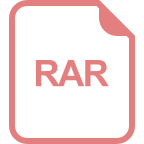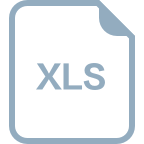# linqjs用法

LinqJS 是一个 JavaScript 库，它提供了类似于 C# LINQ 的查询语言和方法，用于操作数组、集合和对象。以下是 LinqJS 的一些常用用法： 1. 查询数组中的元素： ```javascript var numbers = [1, 2, 3, 4, 5]; var query = Enumerable.from(numbers) .where(function(x) { return x % 2 === 0; }) .toArray(); // 返回 [2, 4] ``` 2. 对数组中的元素进行聚合： ```javascript var numbers = [1, 2, 3, 4, 5]; var sum = Enumerable.from(numbers) .sum(); // 返回 15 ``` 3. 对数组中的元素进行分组： ```javascript var persons = [ { name: '张三', age: 20 }, { name: '李四', age: 30 }, { name: '王五', age: 20 }, { name: '赵六', age: 30 } ]; var groups = Enumerable.from(persons) .groupBy(function(x) { return x.age; }) .toArray(); // 返回 [{ key: 20, values: [{ name: '张三', age: 20 }, { name: '王五', age: 20 }] }, { key: 30, values: [{ name: '李四', age: 30 }, { name: '赵六', age: 30 }] }] ``` 4. 对对象进行查询和转换： ```javascript var persons = { '张三': 20, '李四': 30, '王五': 25 }; var query = Enumerable.from(Object.keys(persons)) .where(function(x) { return persons[x] > 20; }) .select(function(x) { return { name: x, age: persons[x] }; }) .toArray(); // 返回 [{ name: '李四', age: 30 }, { name: '王五', age: 25 }] ``` 以上仅仅是 LinqJS 的一些基本用法，更多的用法可以参考官方文档。

## 相关推荐